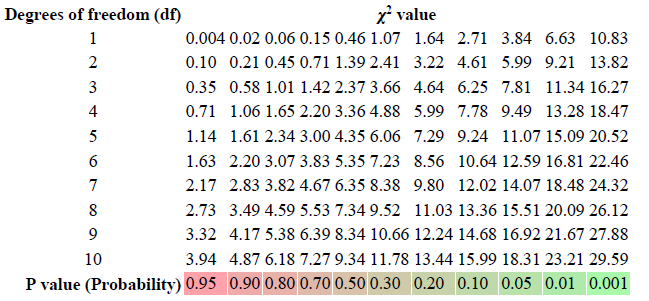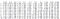# Using sapply() function in R to generate a table

Suppose you want to generate a table for a function f(i,j), for 1 ≤ i ≤ N and 1 ≤j ≤ M. This can be accomplished easily using a double for loop. In a functional programming language like R, the emphasis is to use in-built functions that can perform the task, instead of using a for loop. In this article, I will illustrate how the sapply() function can be used to get the job done using few lines of code.

Suppose we want to reproduce a table of Chi-squared values computed for different p-values as shown on this Wikipedia page: https://en.wikipedia.org/wiki/Chi-squared_distribution:Table of χ2 values vs p-values.

Here is the code in R that does the job:

`p_value <-c(0.95,0.90,0.80,0.70,0.50,0.30,0.20,0.10,0.05,0.01,0.001)DegreeFreedom <-seq(1,10)Chisq_values<-sapply(p_value,function(x)sapply(DegreeFreedom,    function(y)qchisq(1-x, y))) rownames(Chisq_values)=DegreeFreedom colnames(Chisq_values)=p_value print(round(Chisq_values,3))`

Here is the output:Table of χ2 values vs p-values using sapply() function.

As data scientists, it’s important to always make use of functions and libraries in R and python, that way instead of wasting time writing long codes, the focus should be on getting the specific data science project done in a timely and efficient manner.

If you enjoyed reading this article, please clap and share on Twitter and Facebook so that others could benefit as well. Thanks for reading.

Physicist, Data Science Educator, Writer. Interests: Data Science, Machine Learning, AI, Python & R, Personal Finance Analytics, Materials Sciences, Biophysics

## More from Benjamin Obi Tayo Ph.D.

Physicist, Data Science Educator, Writer. Interests: Data Science, Machine Learning, AI, Python & R, Personal Finance Analytics, Materials Sciences, Biophysics